# 3D shapes

Go back to  'Geometry'

 1 Introduction to 3-Dimensional Shapes 2 3-Dimensional Shapes in Mathematics 3 Tips and Tricks 4 Surface Area and Volume of 3D Shapes 5 Properties of 3D Shapes 6 Faces, Edges, and Vertices 7 Examples of 3D Shapes 8 How to Make 3D shapes for Maths project 9 Important Notes on 3D Shapes 10 Solved Examples of 3D Shapes 11 Practice questions on 3D Shapes 12 Maths Olympiad Sample Papers 13 Frequently Asked Questions (FAQs)

We at Cuemath believe that Math is a life skill. Our Math Experts focus on the “Why” behind the “What.” Students can explore from a huge range of interactive worksheets, visuals, simulations, practice tests, and more to understand a concept in depth.

Book a FREE trial class today! and experience Cuemath's LIVE Online Class with your child.

## Introduction to 3-Dimensional Shapes

3D shapes are nothing but solids that have three dimensions - length, breadth, and height.

The D in 3D stands for Dimensional.

These 3D shapes occupy space and are found in our day-to-day life.

We touch, feel and use them.

Some examples of 3D shapes are books, balls, ice cream cones, jugs, donuts etc.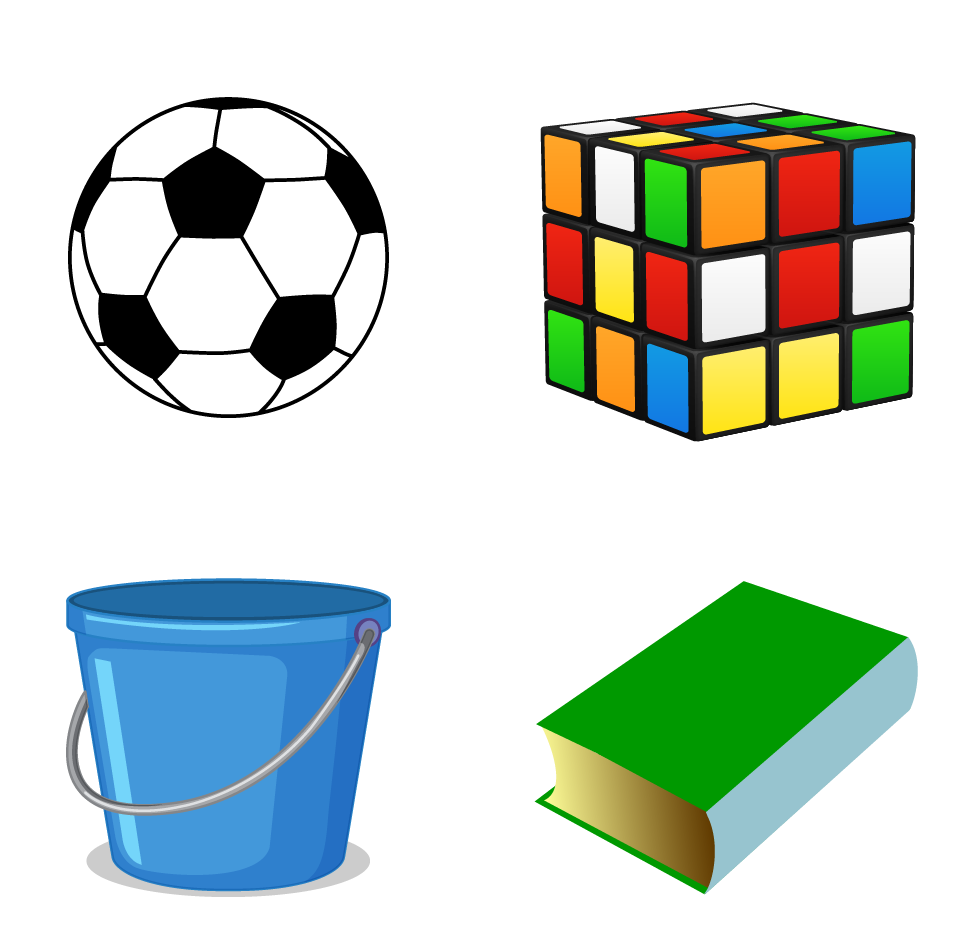## 3-Dimensional Shapes in Mathematics

In mathematics, we study about 3-dimensional objects in the concept of solids and try to apply them in real life.

We will now learn about each 3D shape in detail.

3D shapes are classified into several categories.

Some of them have curved surfaces, pyramids, or prisms.

Let us first learn about the 3-dimensional shapes with curved surfaces.

### Sphere

• It is shaped like a ball and is perfectly symmetrical.
• Every point on the sphere is at an equal distance from the centre.
• It has one face, no edges, and no vertices.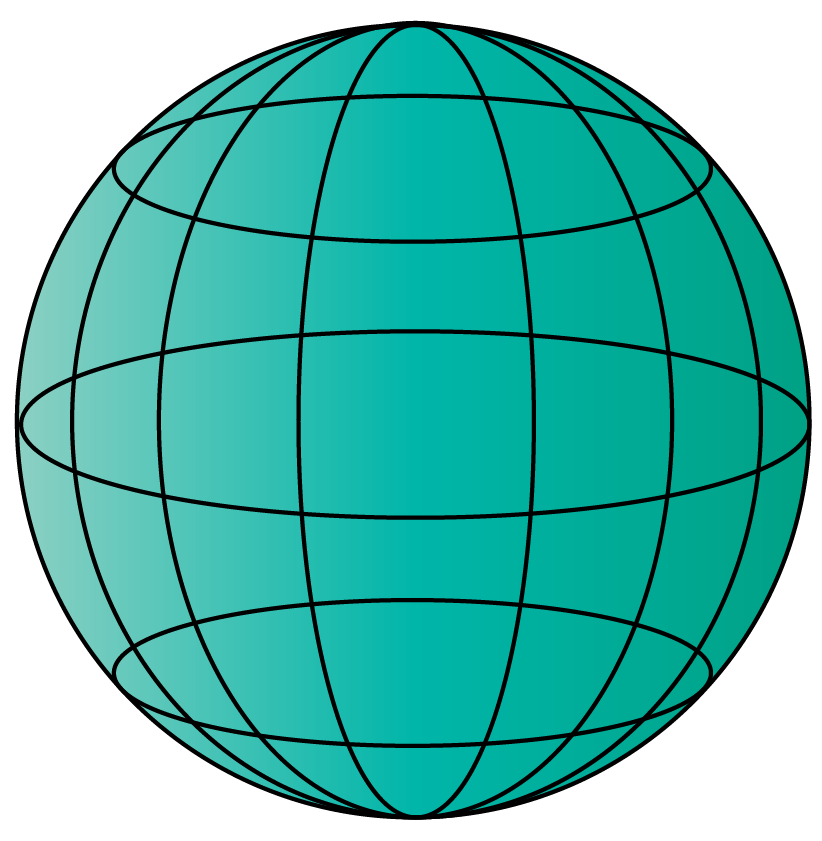### Cylinder

• It has one curved side.
• The shape stays the same from the base to the top.
• It is a three-dimensional object with two identical ends that are either circular or oval.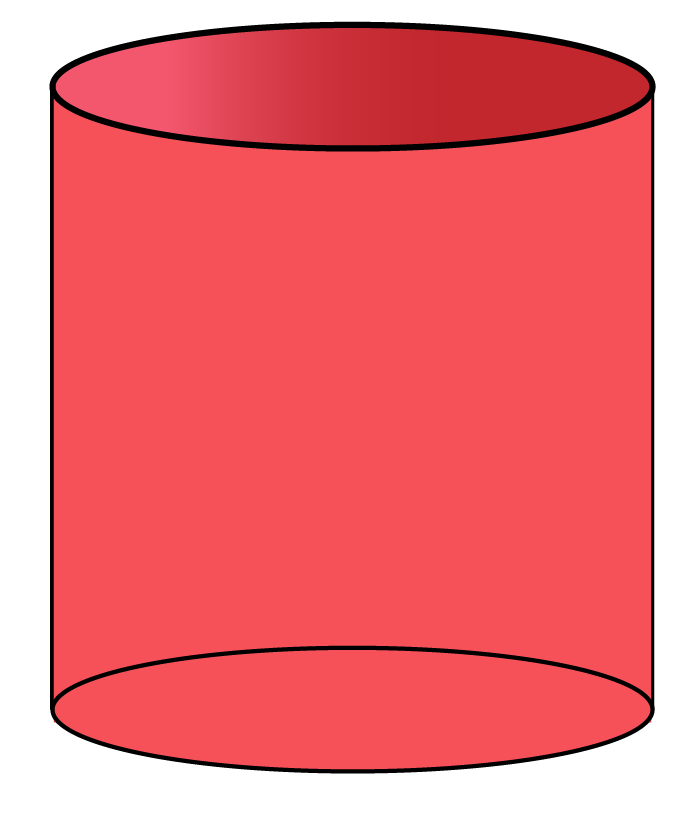### Cone

• A cone has a circular or oval base with an apex (vertex).
• A cone is a rotated triangle.
• Based on how the apex is aligned to the centre of the base, a right cone or an oblique cone is formed.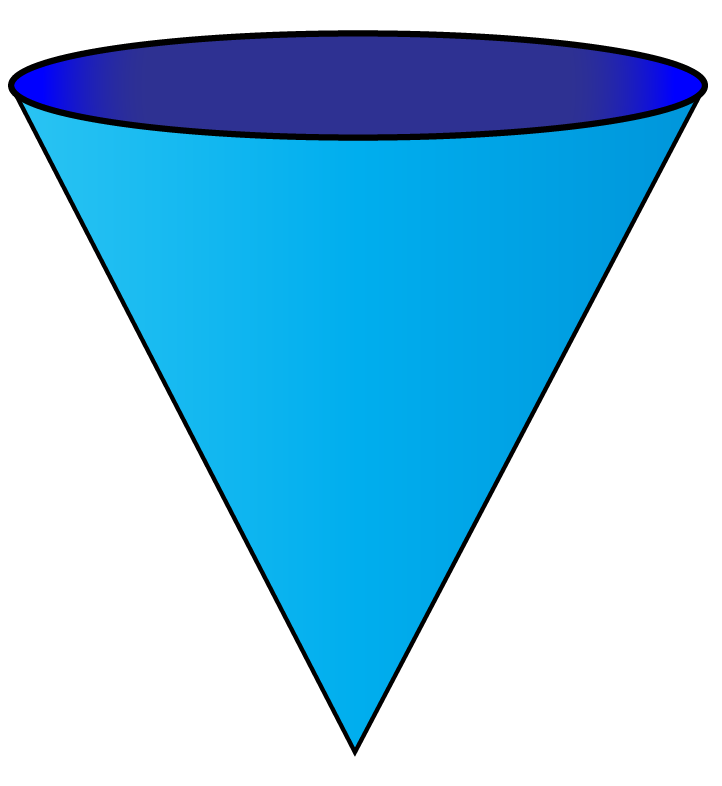### Torus

• A torus is a regular ring, shaped like a tyre or donut.
• It is formed by revolving a smaller circle around a larger circle.
• It has no edges or vertices.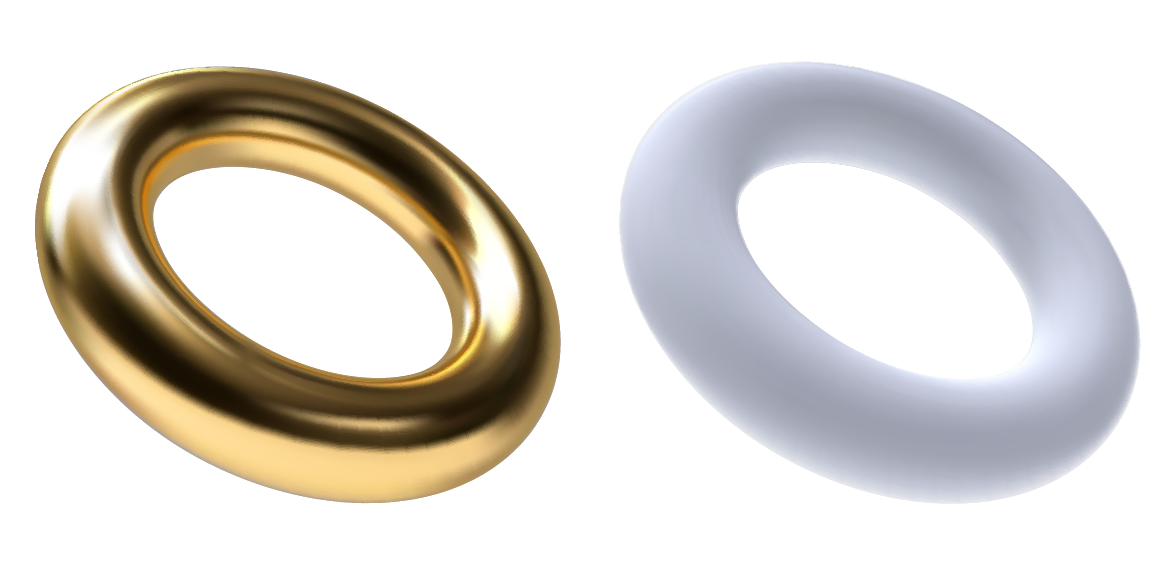Now let us learn about the three dimensional shapes called pyramids.

### Pyramid

• A pyramid is a polyhedron with a polygon base and an apex with straight lines.
• Based on its apex alignment with the centre of the base, they can be classified into regular and oblique pyramids.
• A pyramid with a:
1. Triangular base is called a Tetrahedron
2. Quadrilateral base is called a square pyramid
3. Pentagon base is called a pentagonal pyramid
4. Regular hexagon base is called a hexagonal pyramid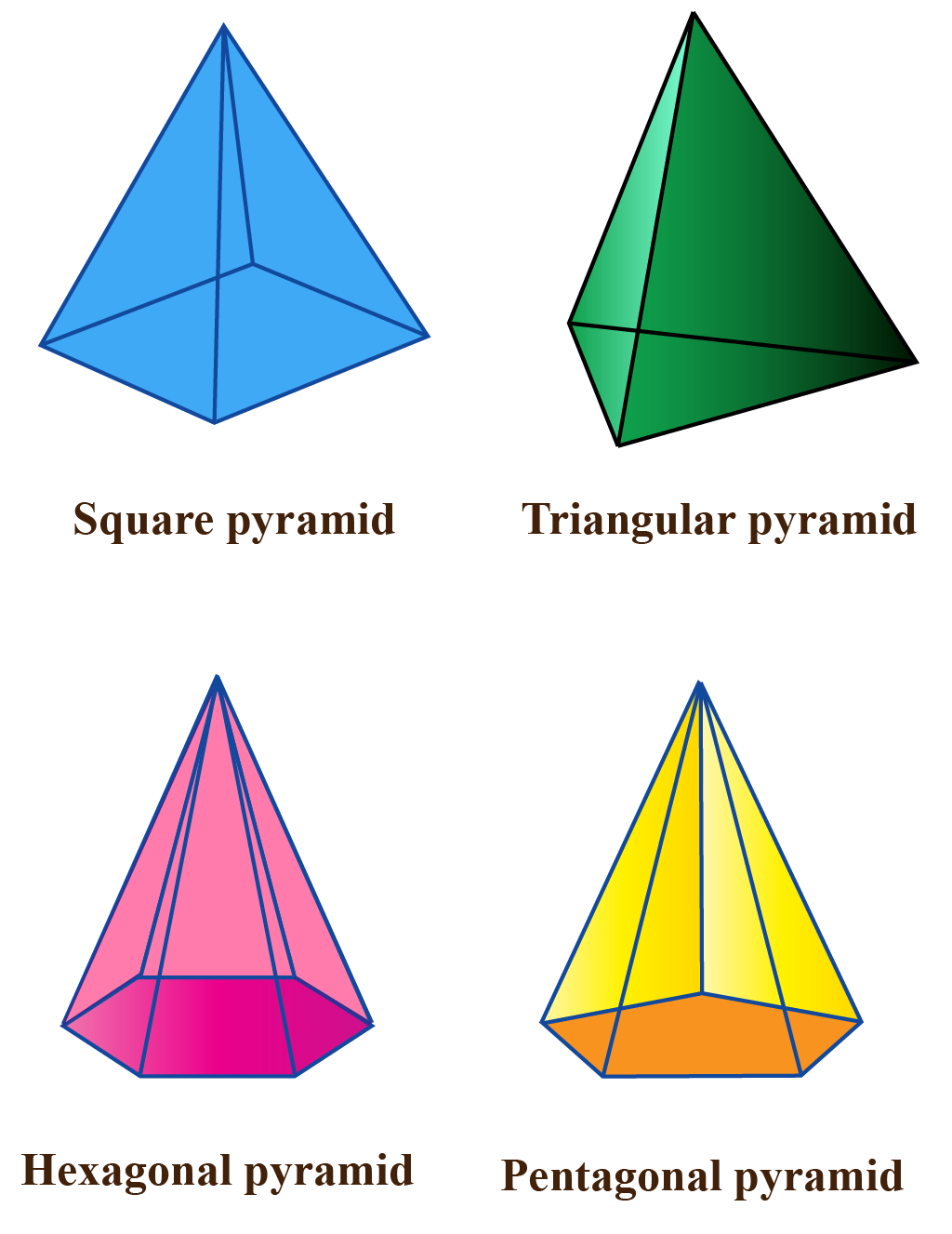Let's learn about the three-dimensional shapes - Prisms.

### Prisms

• Prisms are solids with identical polygon ends and flat parallelogram sides.
• It has the same cross-section all along its length.
• The different types of prisms are - triangular prisms, square prisms, pentagonal prisms, hexagonal prisms, etc.
• Prisms are also broadly classified into regular prisms and oblique prisms.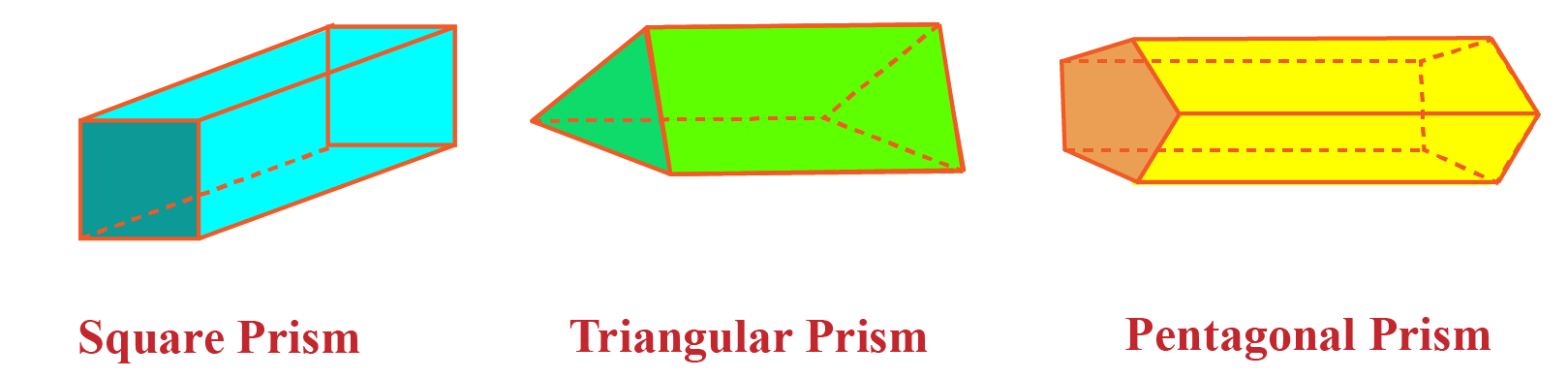Next, let's learn about 3-dimensional shapes with regular polyhedrons (Platonic Solids).

### Polyhedrons / Platonic solids

• There are five polyhedrons.
1. Tetrahedron with four equilateral-triangular faces
2. Octahedron with eight equilateral-triangular faces
3. Dodecahedron with twelve pentagon faces
4. Icosahedron with twenty equilateral-triangular faces
5. Cube with six square faces
• They have identical faces of regular polygons.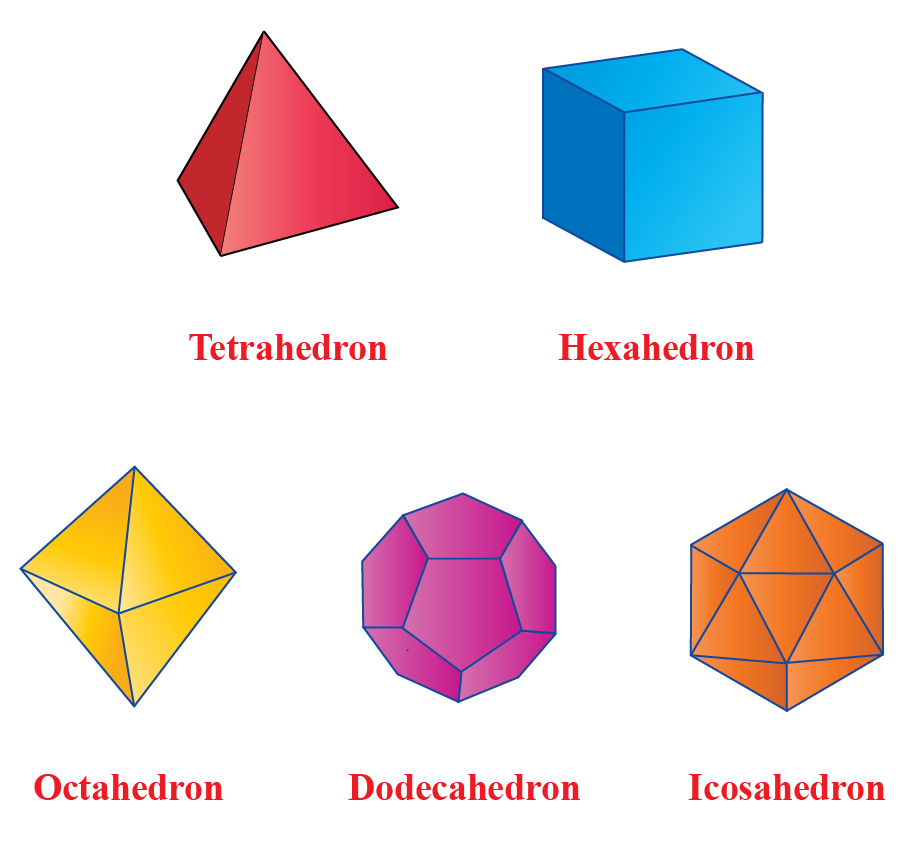## Surface Area and Volume of 3D Shapes

Have you ever wrapped a birthday gift?

When you wrap a gift, you cover the surface area of a cube/cuboid which is a 3-dimensional object.

Similarly, filling a glass by pouring milk into the glass means that you have filled the volume of the glass (cylinder).

We will learn about the surface area and volume of 3D shapes in detail, but before we do that, let's look at simulation.

Move the slider so that you can see the folding and unfolding of wrapping paper for a cuboid-shaped box.

Surface area refers to the area of all the outside surfaces of a three-dimensional object. It is denoted by "SA".

There are three common types of surface areas in 3-dimensional shapes:

• Curved surface area (CSA)
• Lateral Surface area (LSA)
• Total surface area (TSA)

Volume refers to all the space inside a three-dimensional object. It is denoted by "V".

### Surface Area and Volume of 3D Shapes with Curved Surfaces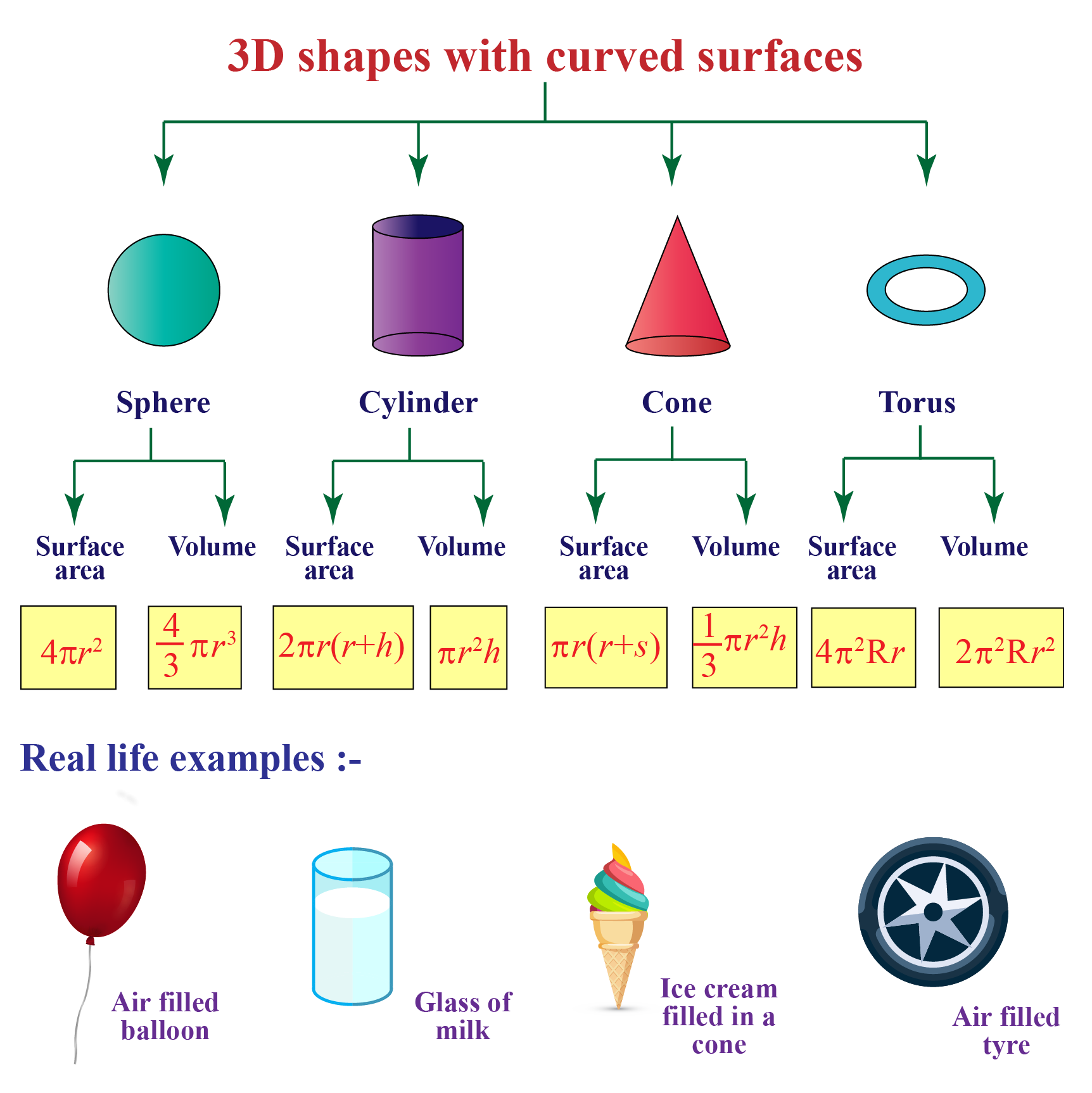### 3-Dimensional Shapes - Pyramids

There are three types of pyramids namely triangular pyramids, square pyramids and pentagonal pyramids.

However, the most common pyramids are square or quadrilateral pyramids.

Surface Area of a Pyramid:

 $$Base\: area + \frac{1}{2} \times (perimeter) \times(slant\: height)$$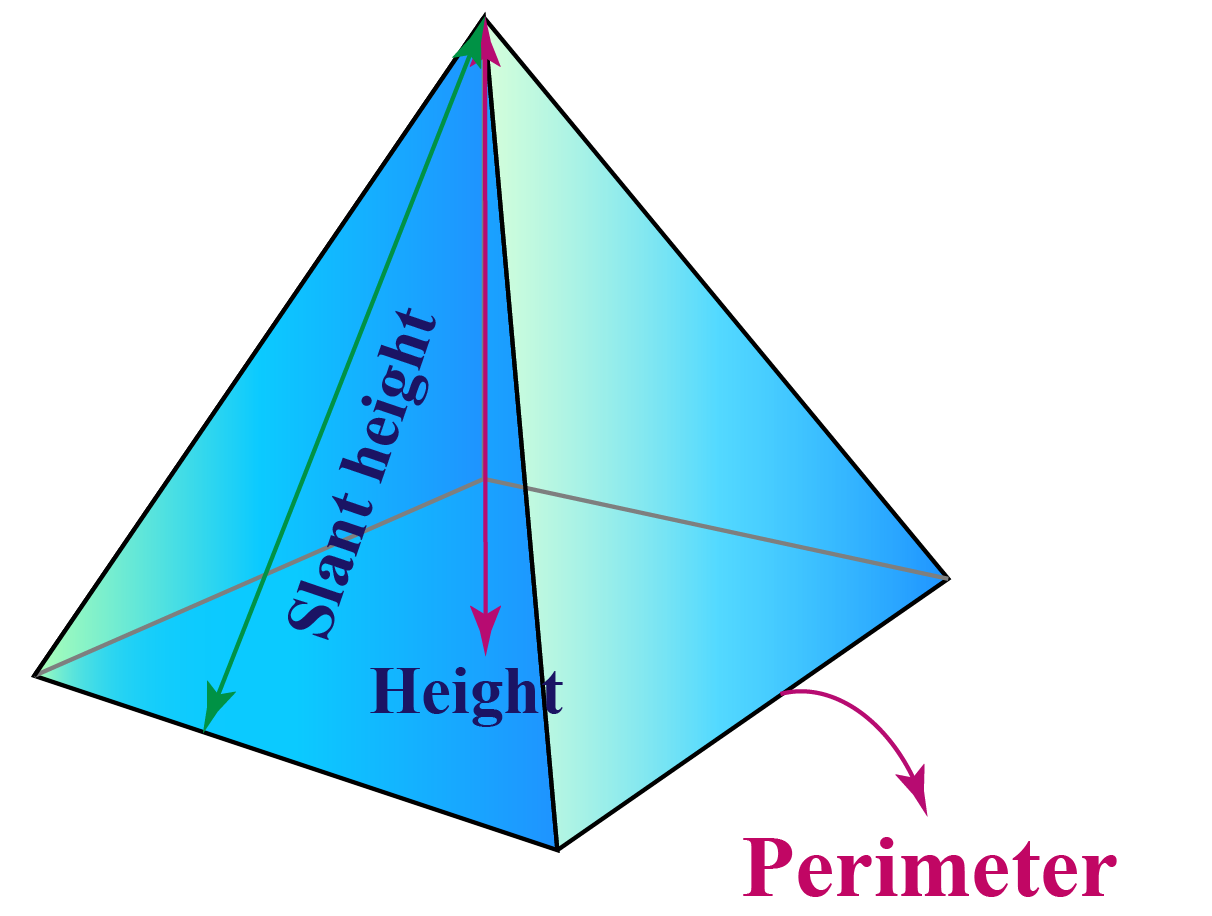Volume of a Pyramid:

 $$\frac{1}{3} \times (Base\: area) \times( height)$$

### 3-Dimensional shapes - Prisms:

Prisms usually have flat faces and their cross-section is the same across its length.

They are found in regular and irregular shapes.

Based on the alignment of the apex to the centre of the base, they are classified as right prisms or oblique prisms.

Surface Area of a Prism

 $$2\times (Base\: area)+ Base\: perimeter \times length$$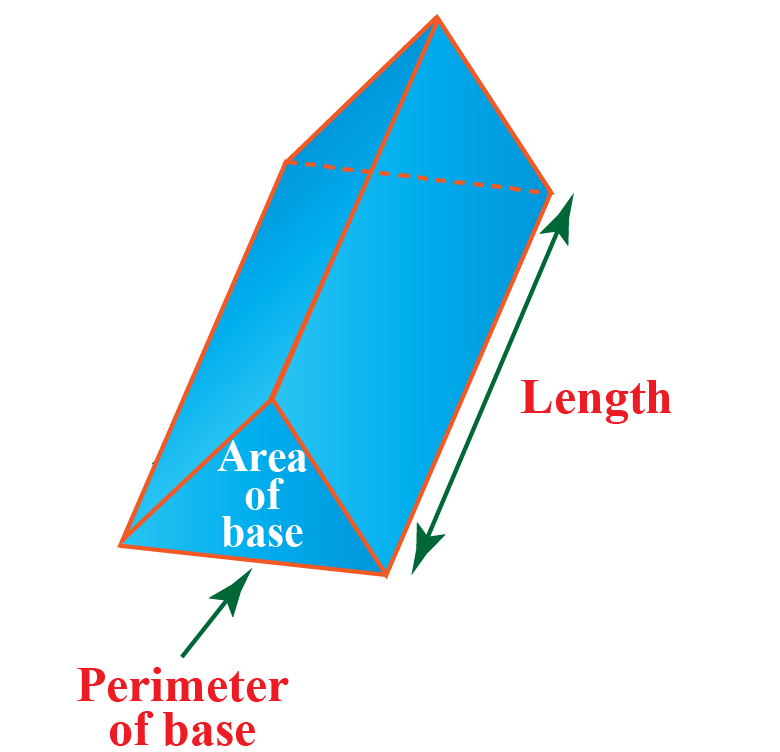Volume of a Prism:

 $$Base\: area \times length$$

## Properties of 3D Shapes

Sphere:

Properties Surface Area Volume
• It has no edges or vertices (corners).
• It has one surface.
• It is perfectly symmetrical.
• All points on the surface are the same distance (r) from the center.
$$4\pi r^2$$ $$\frac{4}{3}\pi r^3$$

Cylinder:

Properties Surface Area Volume
• It has a flat base and a flat top.
• The bases are always congruent and parallel.
• It has one curved side.
$$2\pi r(r+h)$$ $$\pi r^2h$$

Cone:

Properties Surface Area Volume
• It has a flat base.
• It has one curved side.
$$\pi r(r+s)$$ $$\sqrt{3}\pi r^2h$$

Cube:

{Where edge length (e L)}

Properties Surface Area Volume
• It has 6 faces, each with 4 edges (and is a square).
• It has 12 edges.
• It has 8 vertices (corner points) where 3 edges meet.
$$6 \times (e\: L)^2$$ $$(e\: L)^3$$

Prism:

{Where base area (B A), Perimeter (P), Length (L)}

Properties Surface Area Volume
• It has identical ends (polygonal) and flat faces.
• It has the same cross-section all along its length.
$$2\times (B\: A)$$+ $$B\:P \times L$$ $$B\: A\times L$$

Pyramid:

{Where base area (B A), Perimeter (P), Slant Height (S H)

Properties Surface Area Volume
• It is a polyhedron with a polygon base and an apex with straight lines.
• Based on its apex alignment with the center of the base, they can be classified into regular and oblique pyramids.

$$B\:A$$ + $$\frac{1}{2} \times P \times(S\: H)$$

$$\frac{1}{3} \times B\: A \times H$$

## Faces, Edges, and Vertices

As mentioned before, 3D shapes and objects are different from 2D shapes and objects because of the presence of the three dimensions - length, breadth, and height.

As a result of these three dimensions, these objects have faces, edges, and vertices.

Let's understand these three in detail.

### Faces of 3D Shapes

• A face refers to any single flat surface of a solid object.
• 3D shapes can have more than one face.

### Edges of 3D Shapes

• An edge is a line segment on the boundary joining one vertex (corner point) to another.
• They serve as the junction of two faces.

### Vertices of 3D Shapes

• A point where two or more lines meet is called a vertex.
• It is a corner.
• The point of intersection of edges denotes the vertices.

For example: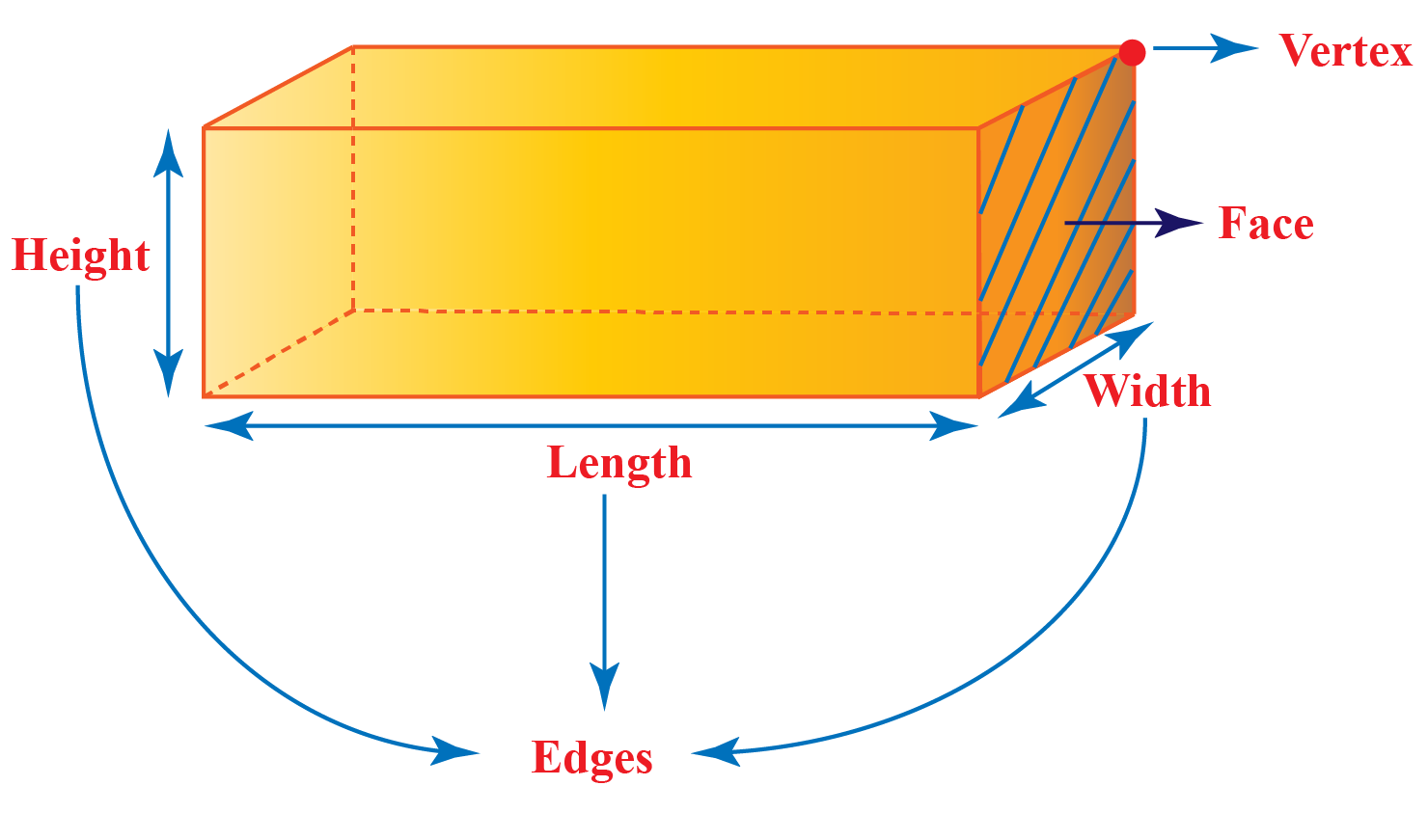3 D shapes Faces Edges Vertices
Sphere

1

0 0
Cylinder

2 0
Cone

2

2 1
Cube

6

12 8
Rectangular Prism

6

12 8

Triangular Prism

5 9 6

Pentagonal Prism

7 15 10

Hexagonal Prism

8 18 12

Square Pyramid

5 8 5

Triangular Pyramid

4

6 6

Pentagonal Pyramid

6 10 6

Hexagonal Pyramid

7 12 7

## Examples of 3D Shapes

To summarize the difference between 2D and 3D objects, we can say that 2-dimensional objects are flat while 3-dimensional objects have depth in them.

Look at the table below to distinguish between 2D and 3D shapes and objects.

2D Shapes 3D Objects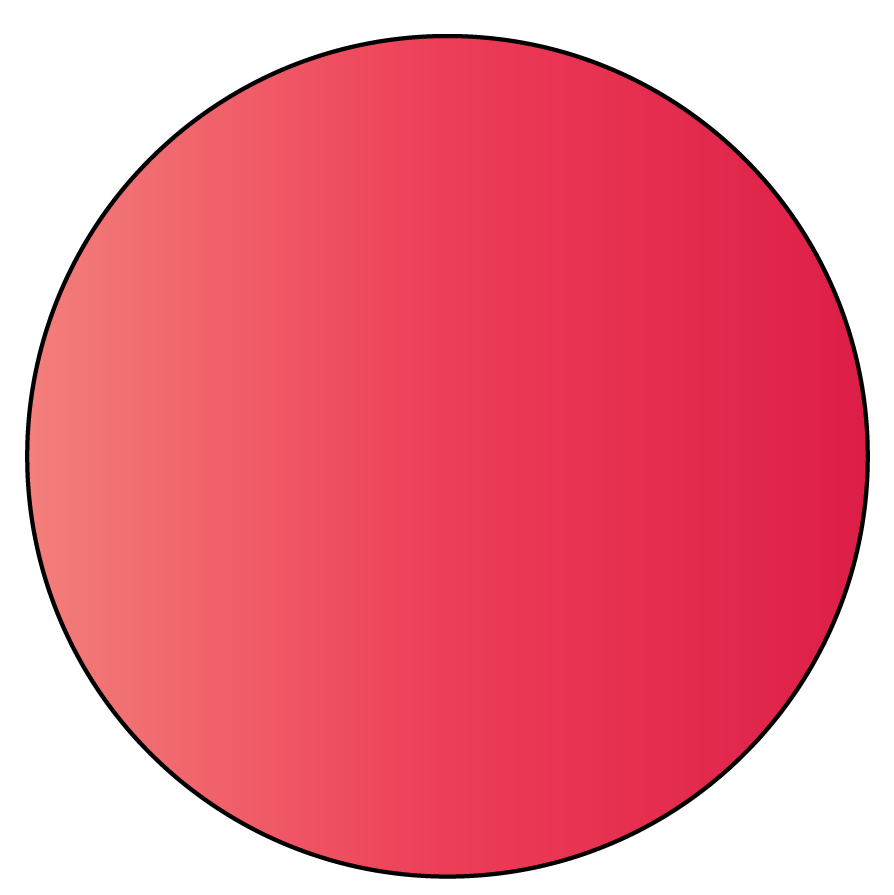Circle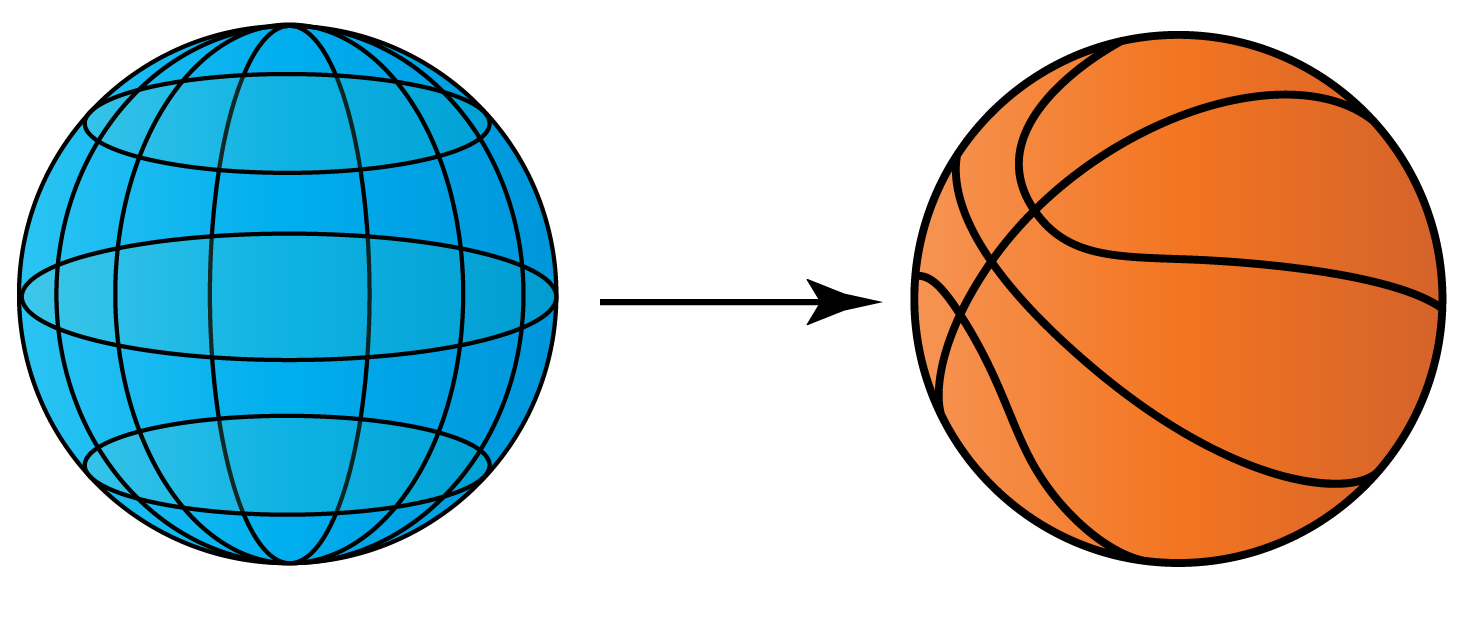-

Sphere - Ball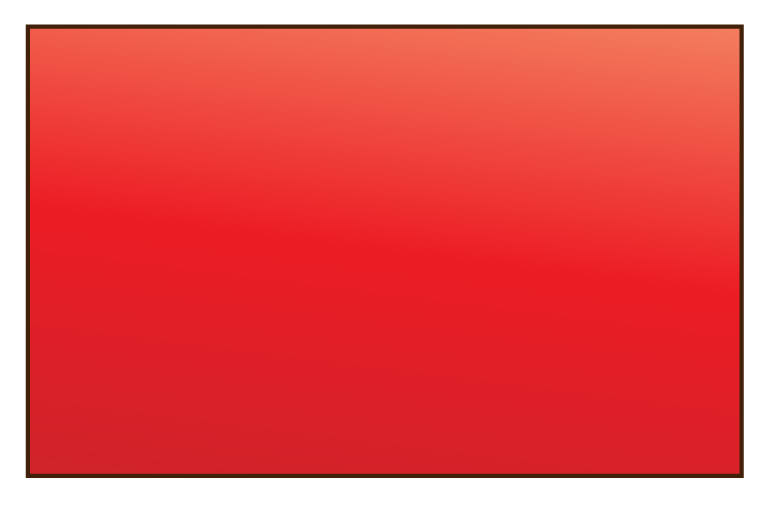Rectangle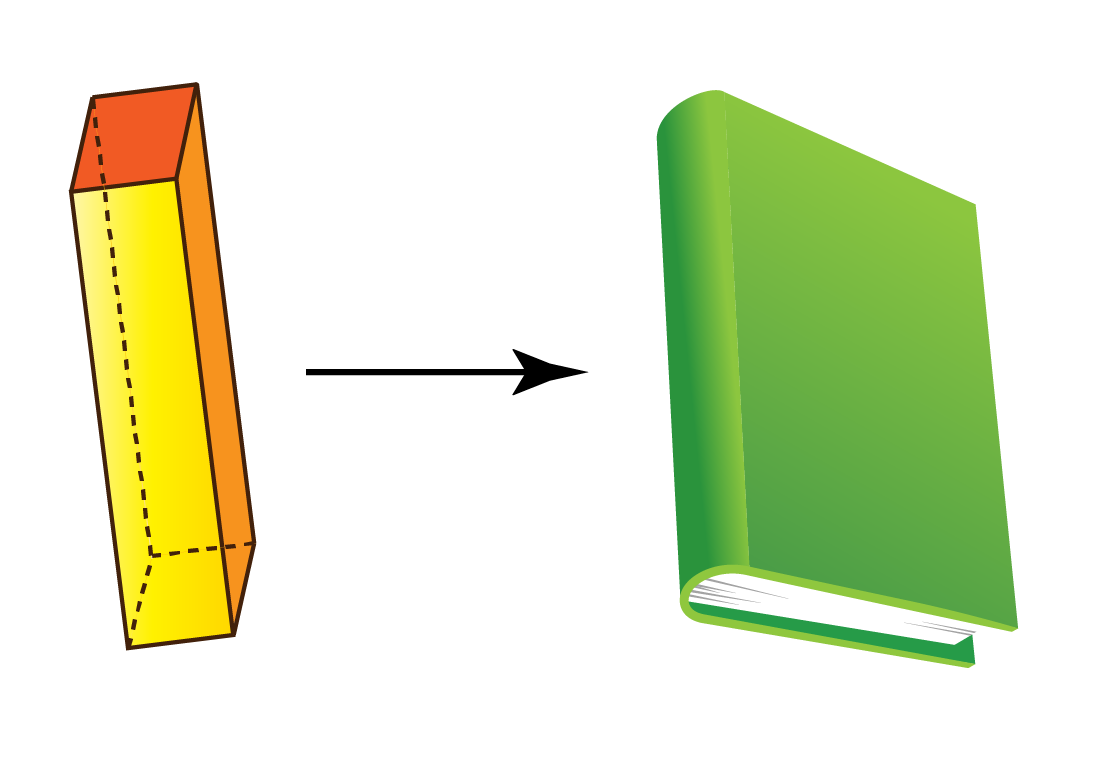Rectangular prism - Book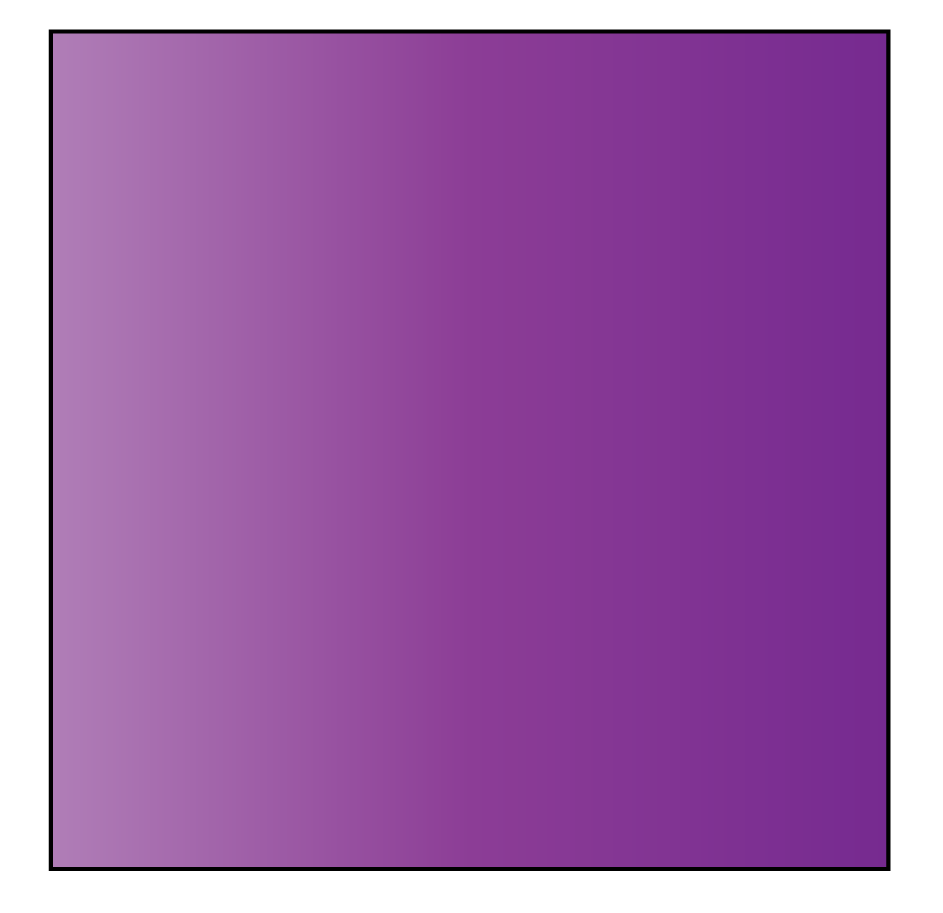Square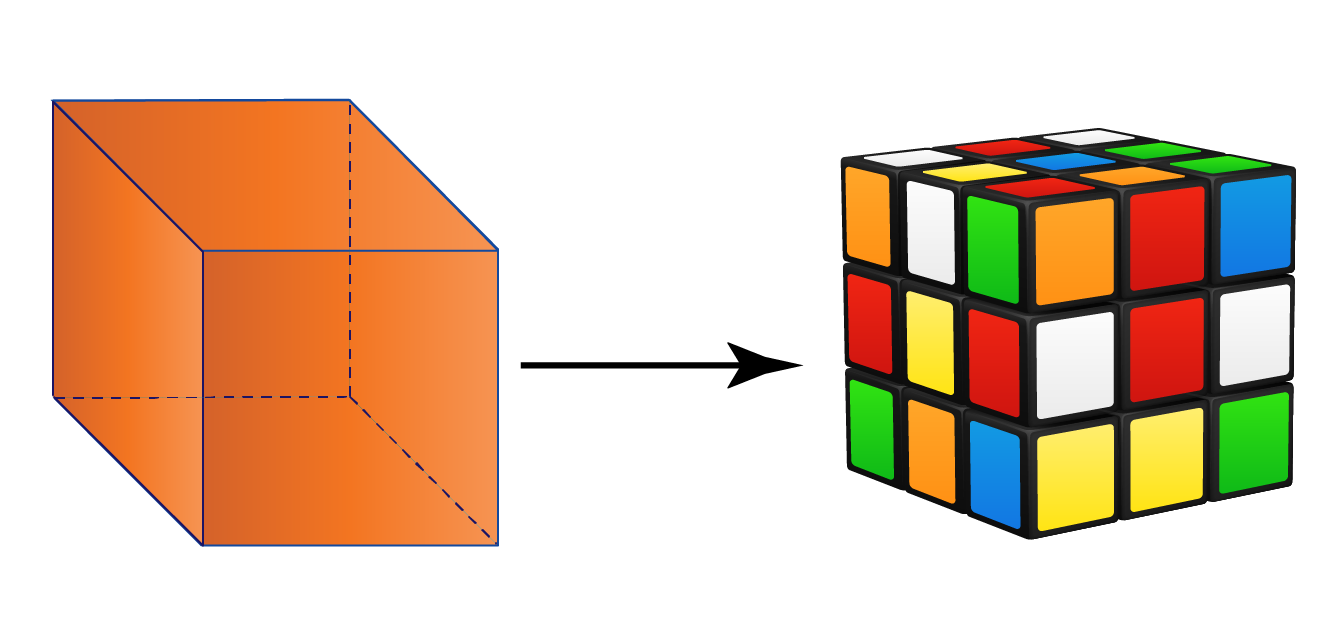Cube - Rubik's cube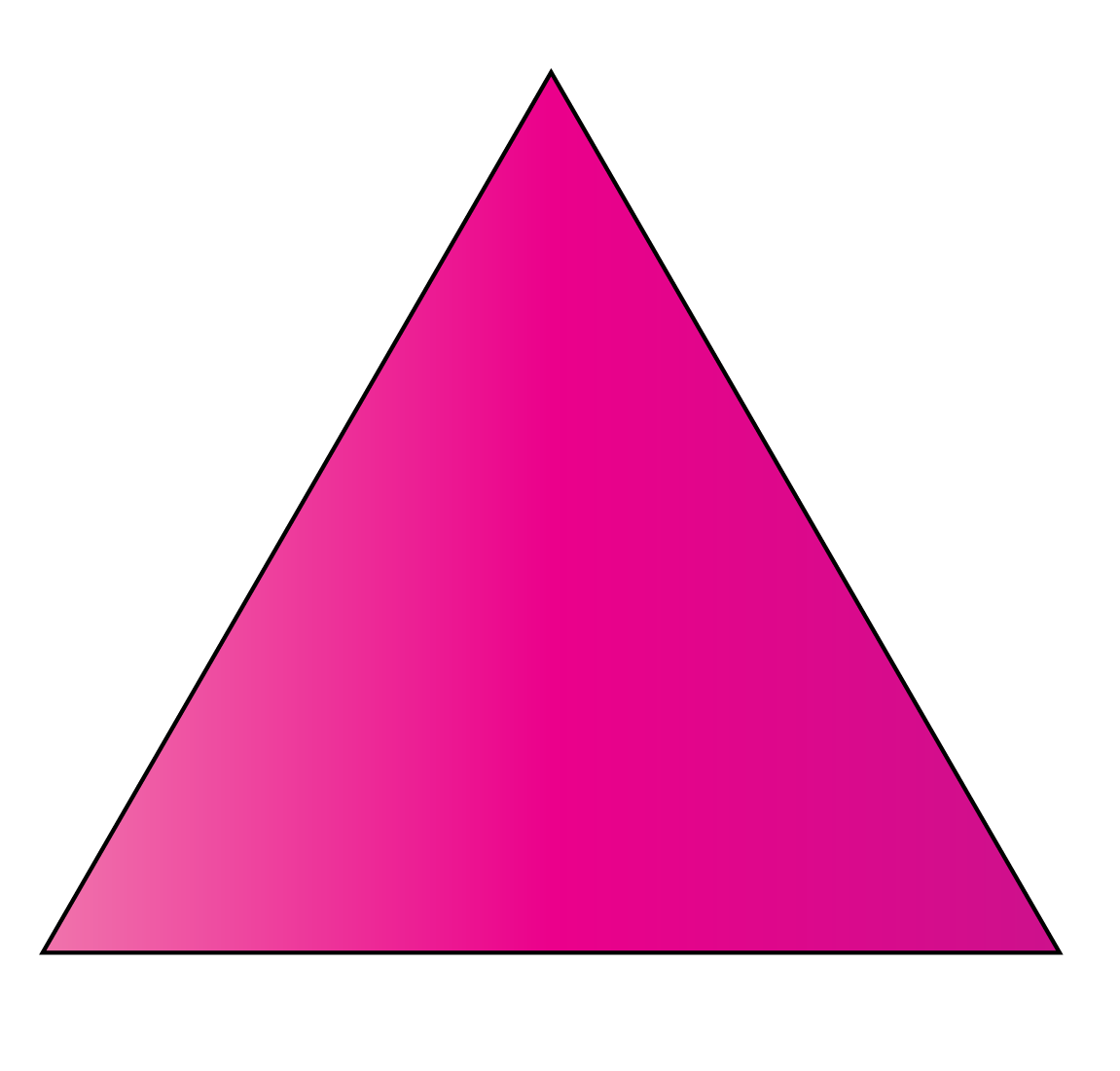Triangle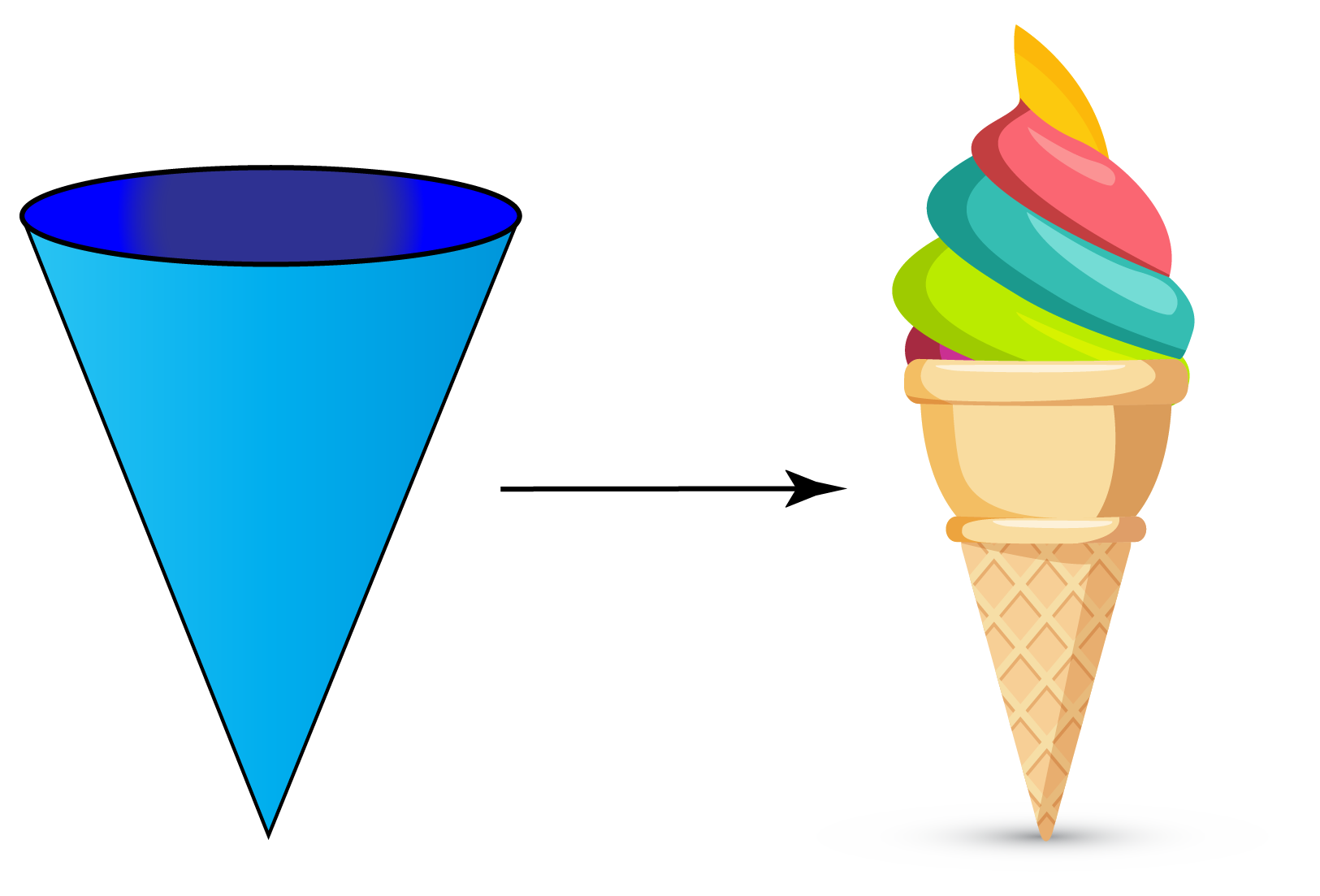Cone - Ice cream cone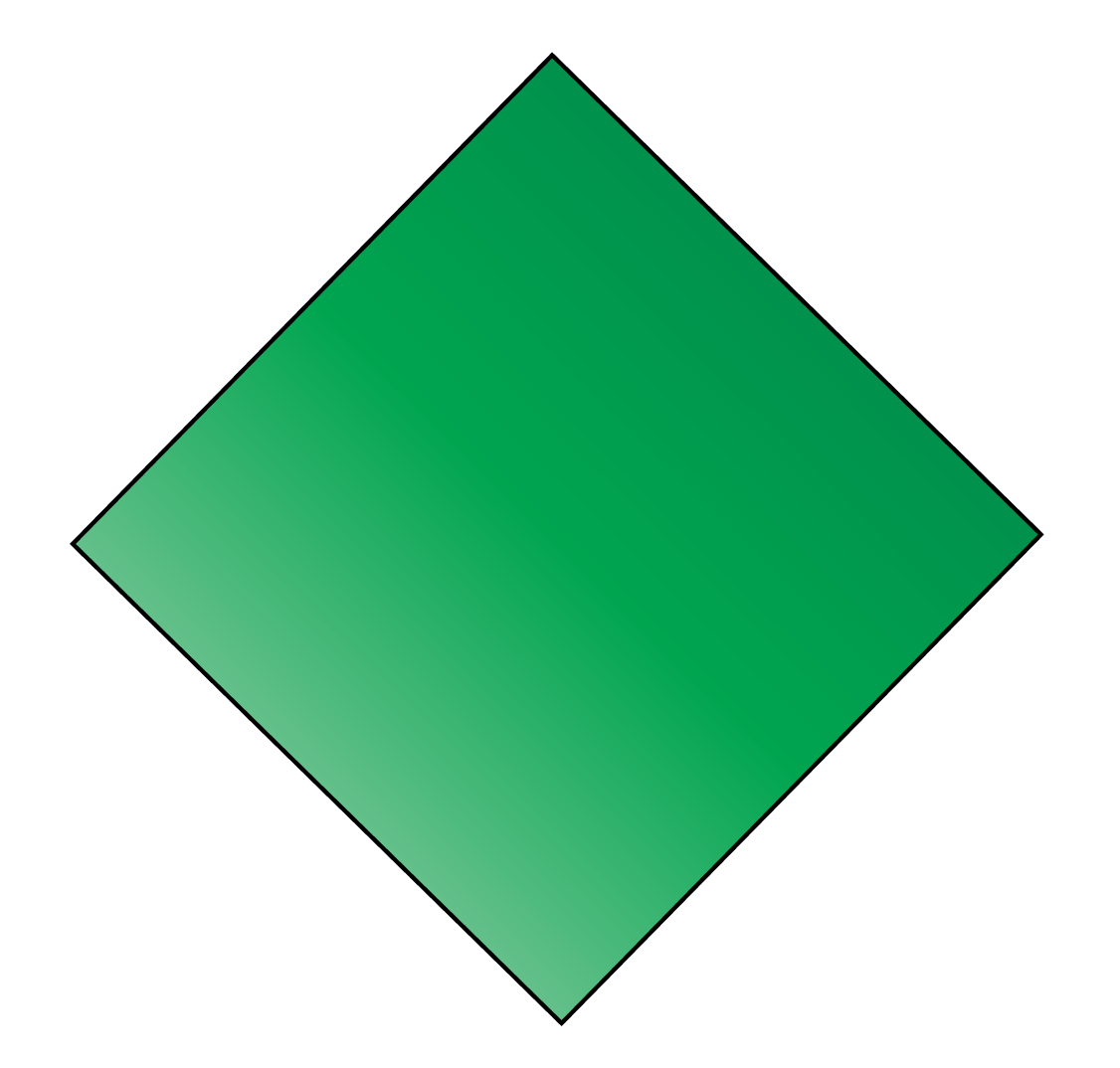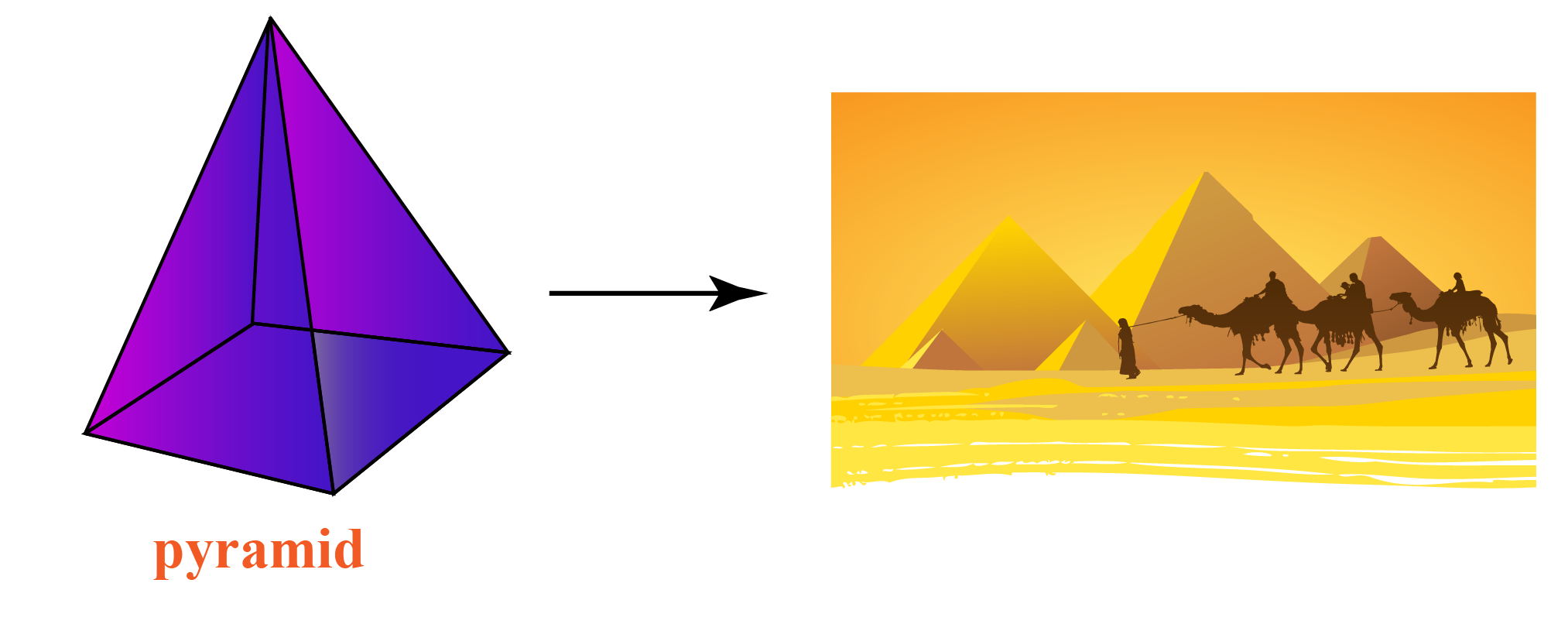Pyramid - Pyramids in Egypt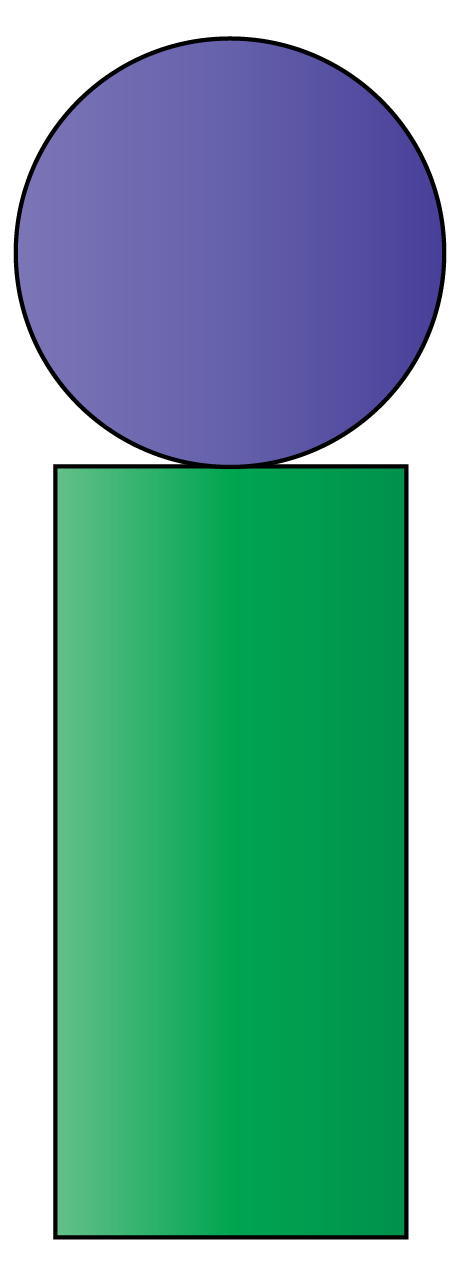Rectangle and circle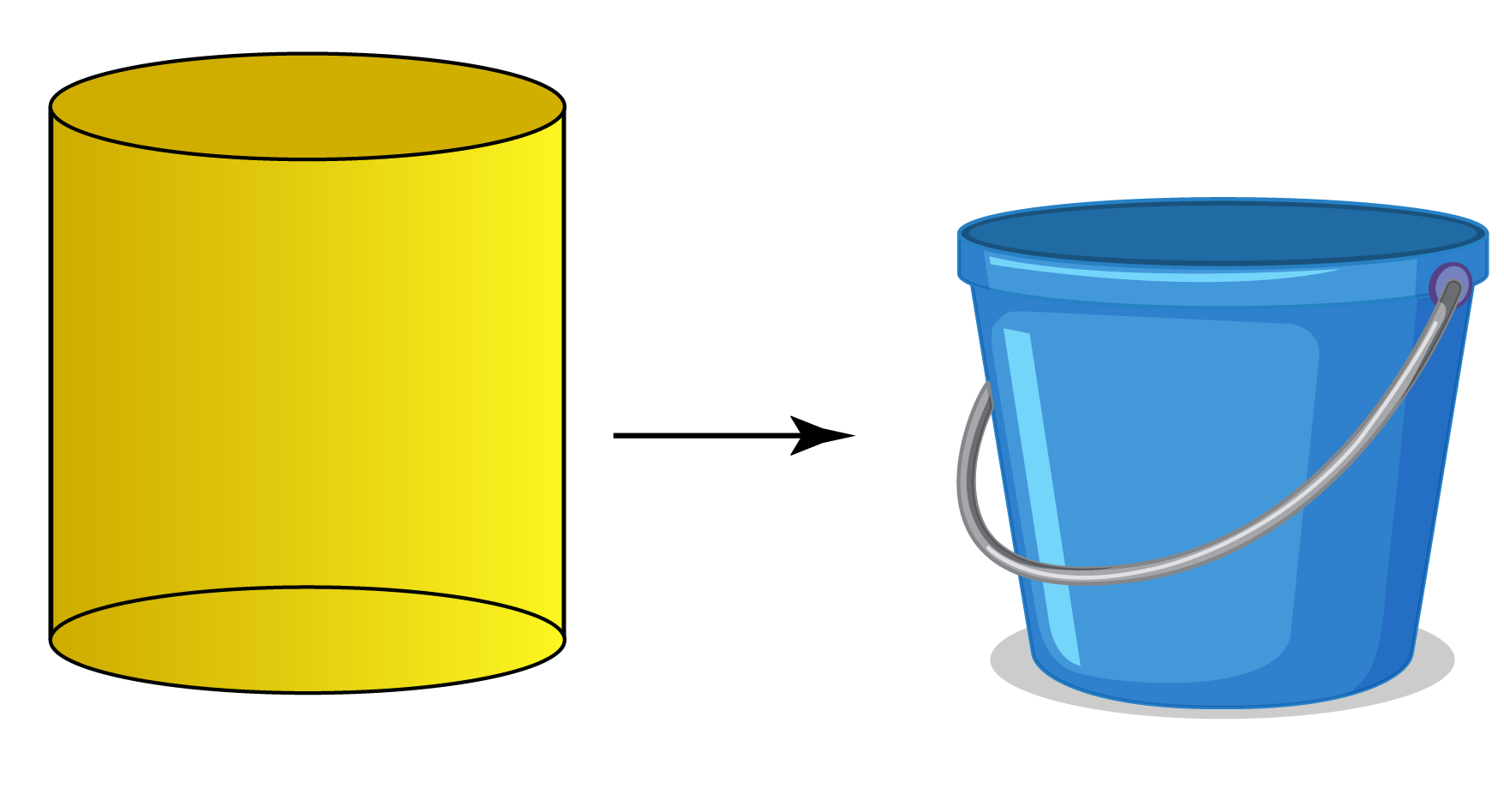Cylinder - Bucket

## How to make 3D Shapes for Math Projects

We can make 3D shapes for our math projects using geometrical nets.

A 2-dimensional shape that can be folded to form a 3-dimensional object is known as a geometrical net.

A solid may have different nets.

In simple words, the net is an unfolded form of a 3D figure.

Let's practice making 3D shapes using the simulations shown below.

Move the slider to see how the nets shape into three-dimensional figures.

The following simulation shows 3D shapes with curved surfaces.

Move the slider and the screen to explore the view from different angles.

The next simulation shows how to create 3D shapes which have the properties of a regular polyhedron.

You can create your own nets with a piece of paper by using the templates given beneath each shape.

##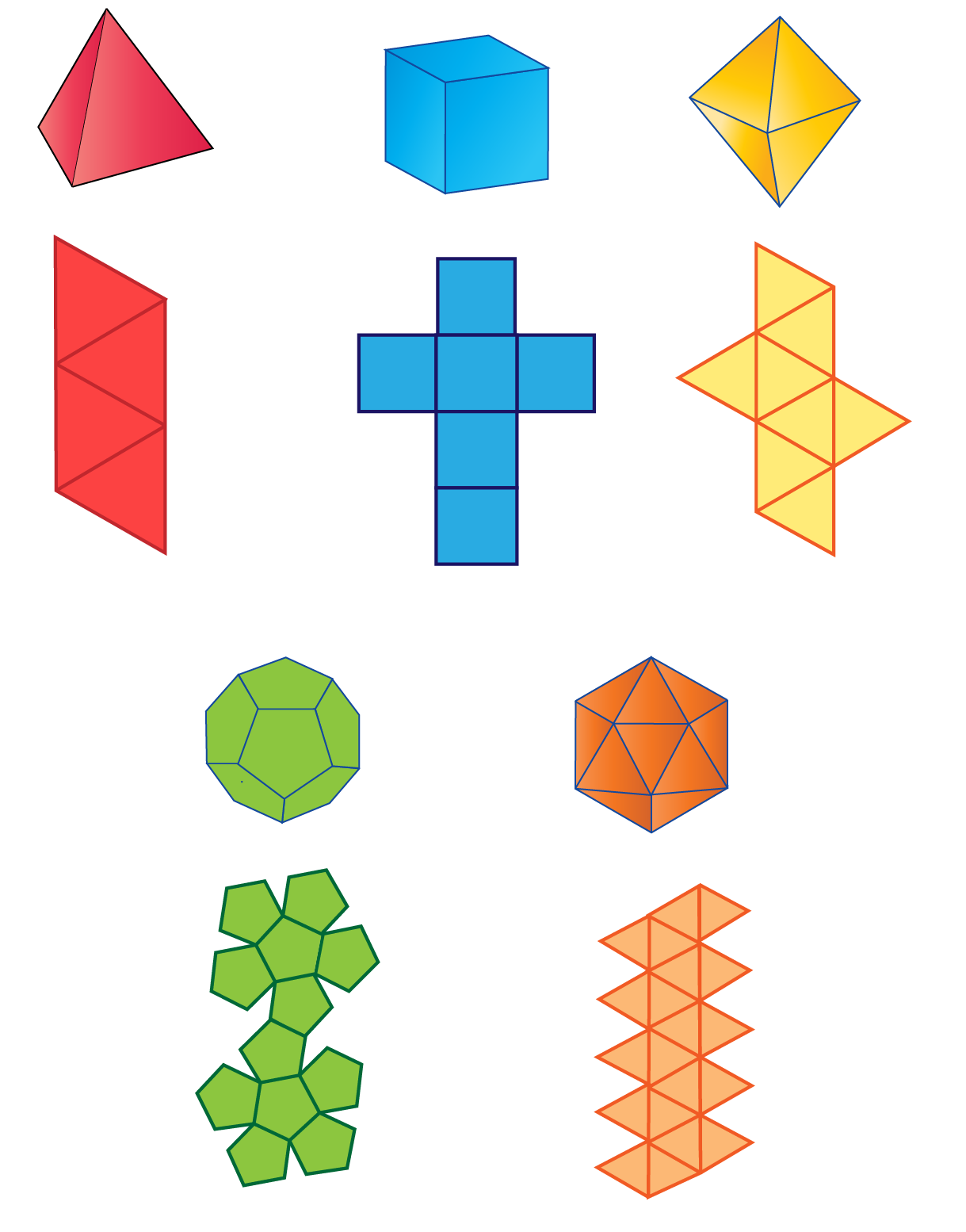Important Notes
1. Three-dimensional objects have 3 dimensions namely length, breadth and height.
2. 3D shapes have faces, edges and vertices.
3. Surface area is classified into:
1. Curved surface area
2. Lateral surface area
3. Total surface area
4. Learning about 3D solids will help us in our day to day life as most of our activities revolve and depend on them.

Help your child score higher with Cuemath’s proprietary FREE Diagnostic Test. Get access to detailed reports, customized learning plans, and a FREE counseling session. Attempt the test now.

## Solved Examples on 3D Shapes

 Example 1

A mason wants to build a 3D sphere using cement.

He wants to know the amount of cement required to construct the sphere of radius 10 cm.

Find the volume of the sphere using the given radius.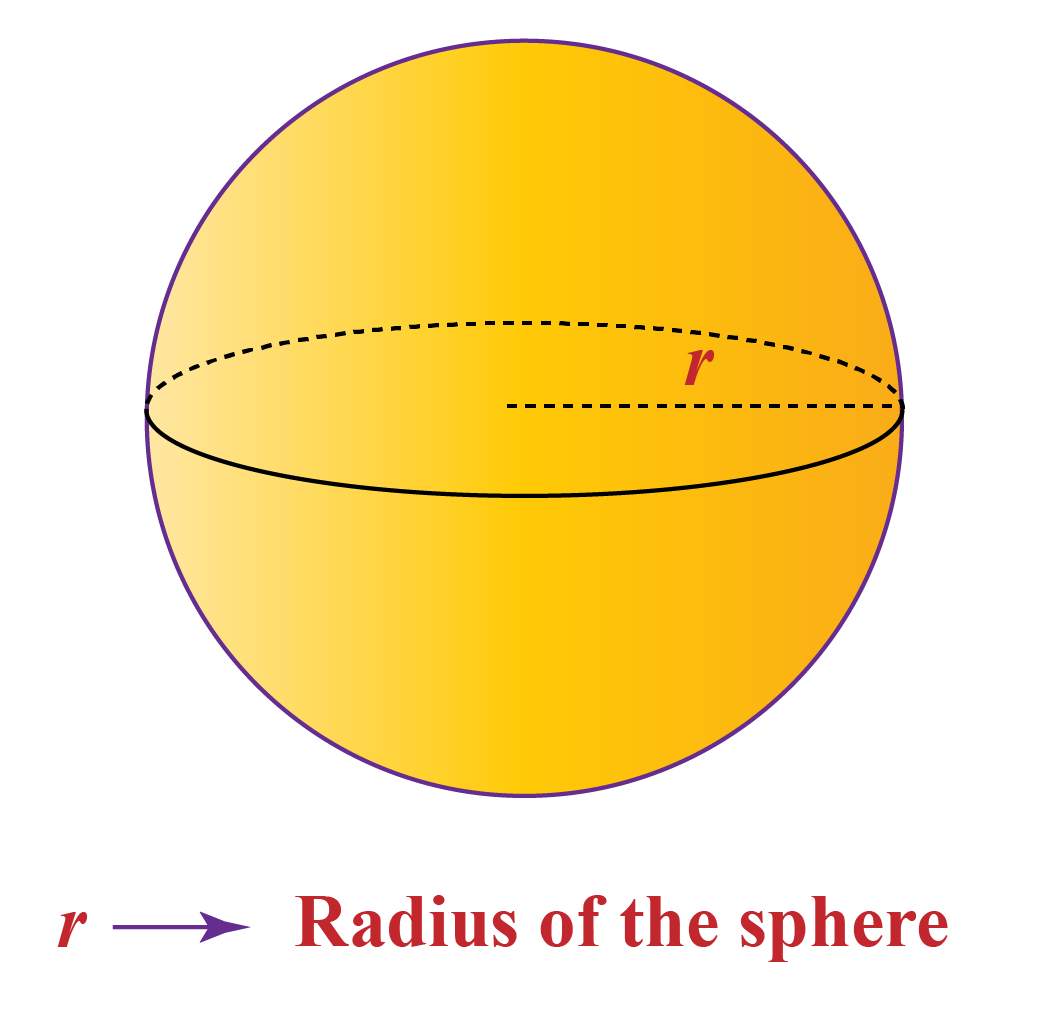Solution:

Given,

The radius of the sphere (r) = 10 cm

We know the formula for the volume of a sphere:

 \begin{align}v=\frac{4}{3}\pi\ r^3 \end{align}

The volume of the cement sphere $$v=\frac{4}{3}\pi\ r^3$$

Substituting the value of the radius in the above formula, we get:

$$v=\frac{4}{3}\pi\ r^3$$

$$v=\frac{4}{3}\pi (10)^3$$

$$v= 4188.8 \: cm^3$$

 $$\therefore$$ volume of the cement sphere is $$4188.8\: cm^3$$
 Example 2

Find the surface area of a cuboid of length 3 cm, breadth 4 cm, and height 5 cm.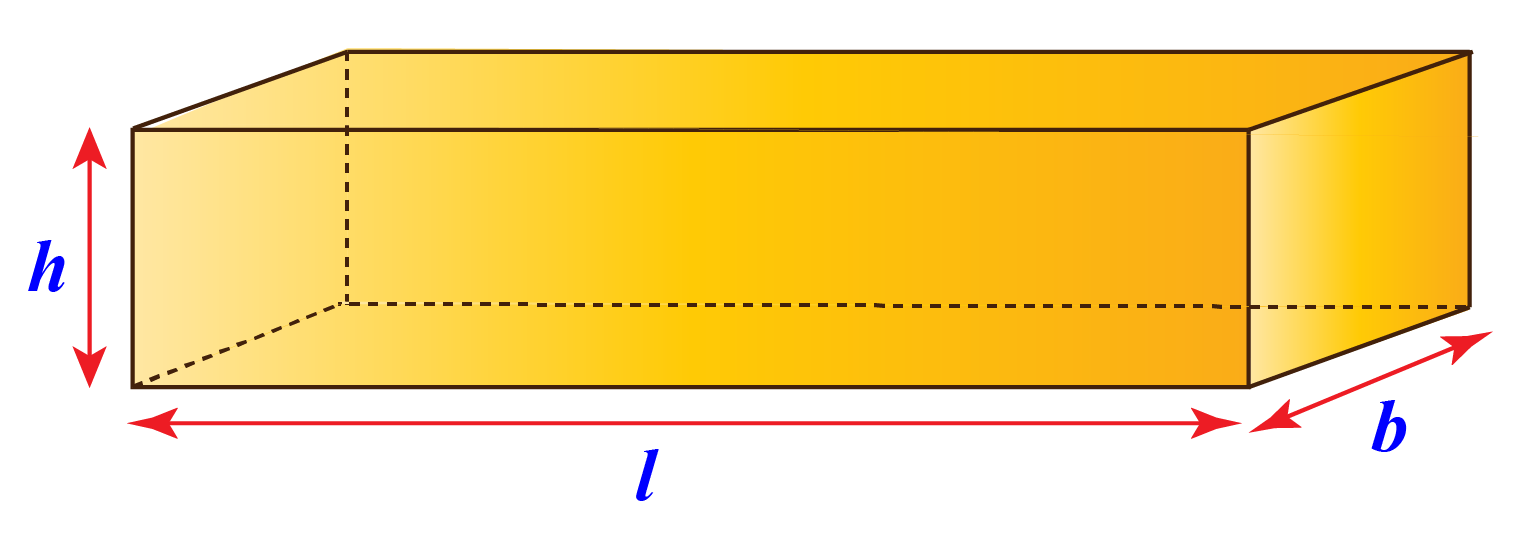Solution:

Given that,

Length of the cuboid = 3 cm

Breadth of the cuboid = 4 cm

Height of the cuboid = 5 cm

Surface area of the cuboid is

 \begin{align}2 \times (lb + bh + lh) \end{align}

$$= 2 \times (lb + bh + lh)$$

$$= 2(3\times 4 + 4 \times 5+ 3\times 5$$)

$$= 2(12+20+15$$)

$$=2(47)$$

$$=94 \: cm^2$$

 $$\therefore$$ Surface Area of cuboid = $$94\: cm^2$$
 Example 3

Bhavya wants to drink milk in a glass which is in the shape of a cylinder.

The height of the glass is 15 cm and the radius of the base is 3 cm.

What is the quantity (volume) of milk that she requires to fill the glass?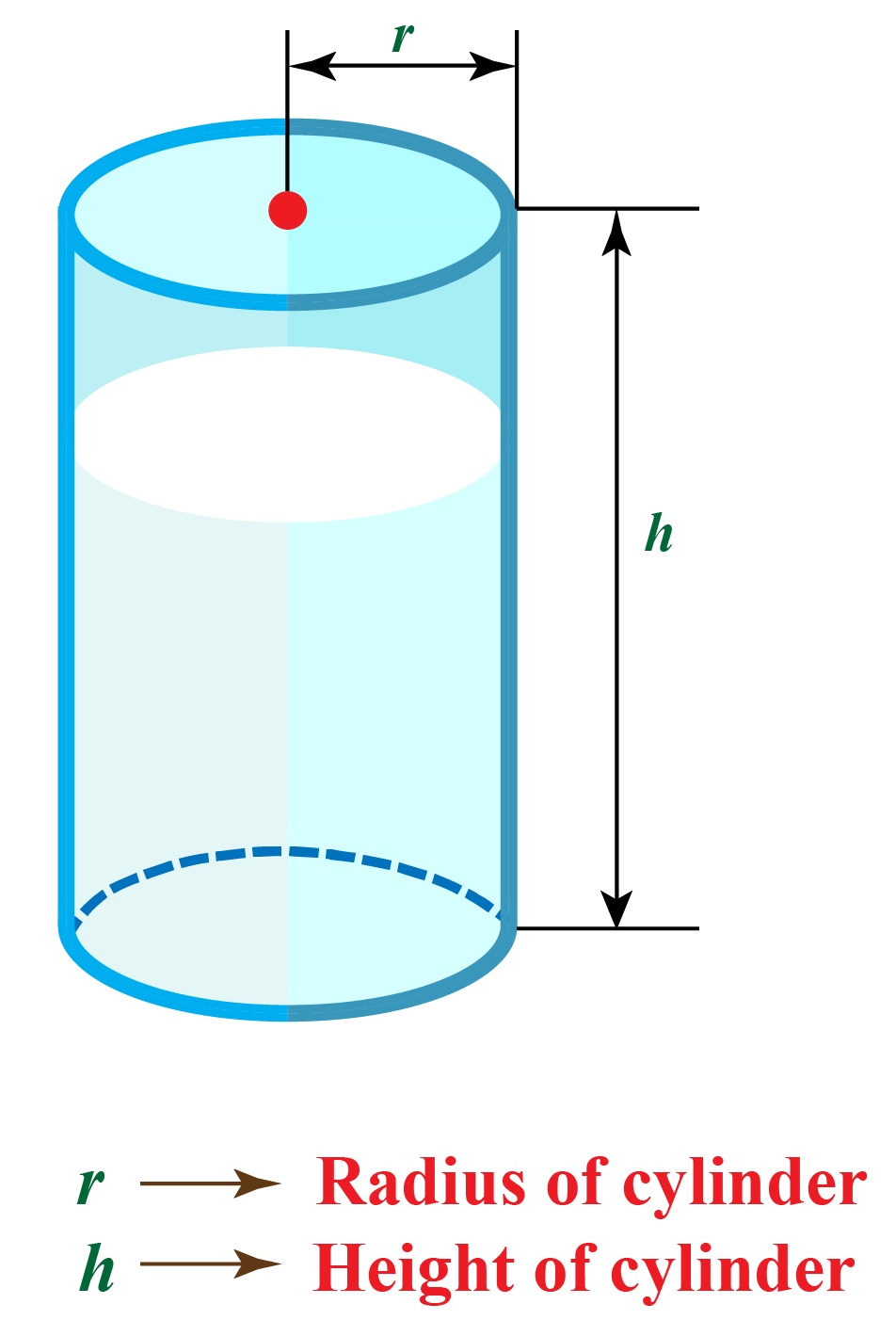Solution:

Given that,

Height of the glass  = 15 cm

Radius of the glass base = 3 cm

To find the volume of the glass, we need to use the formula for the volume of a cylinder, which is

 \begin{align}\pi r^2h \end{align}

The volume of the glass, V = $$\pi r^2h$$

$$V= \pi (3)^2 \times 15$$

$$V= \pi (135)$$

$$V= 424.11\:cm^3$$

Therefore, she needs roughly 425 $$cm^3$$ of milk to fill her glass.

 $$Volume =424.11\: cm^3$$
 Example 4

Identify the regular polyhedron from the images shown below.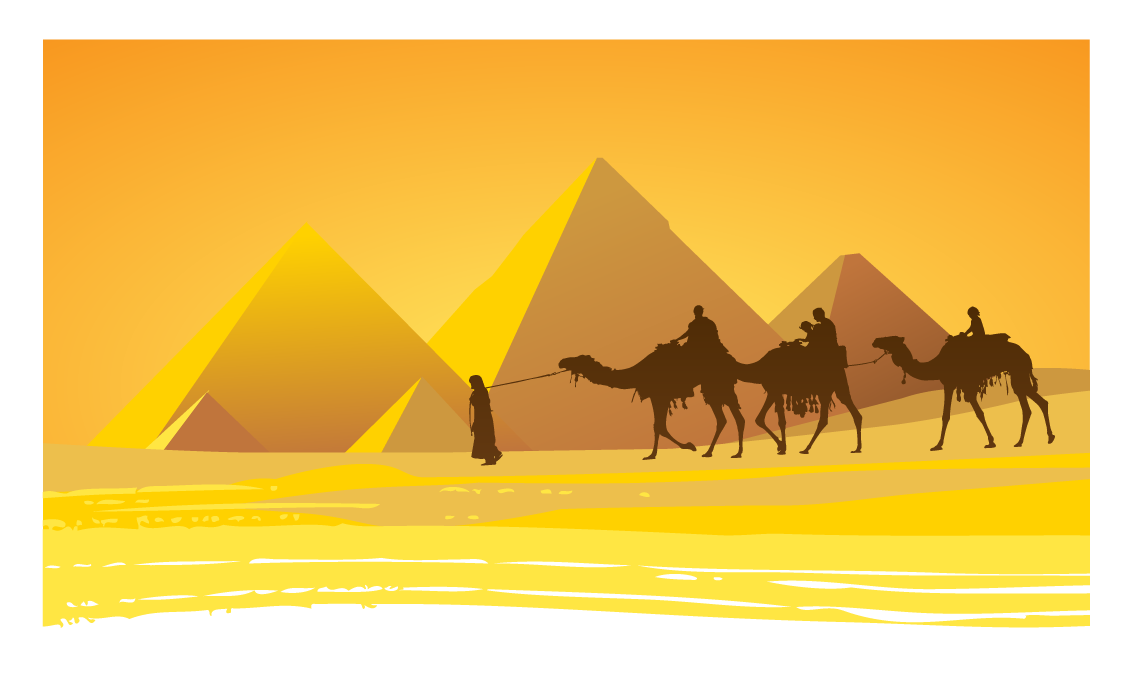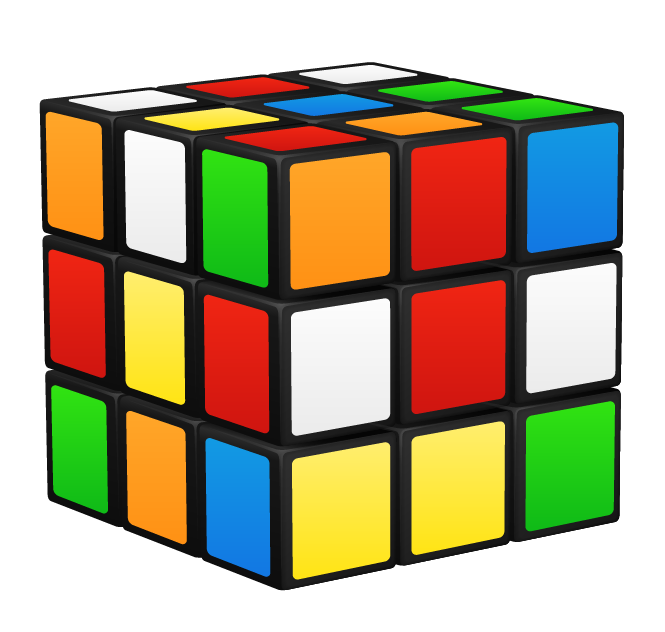Solution:

Regular polyhedrons include:

1. Prisms
2. Pyramids
3. Platonic solids

The given examples of polyhedron must come under these categories.

Thus, the Egyptian pyramids and Rubik's cube are polyhedrons.

 $$\therefore$$ Egyptian Pyramids and Rubik's Cube
 Example 5

Give a few examples of real-life objects in the shape of TORUS.

Solution:

1. Donut
2. Car Tyres
3. Ring
4. Polo (mint freshener candy)
5. Swimming tube and so on...

CLUEless in Math? Check out how CUEMATH Teachers will explain 3D Shapes to your kid using interactive simulations & worksheets so they never have to memorise anything in Math again!

Explore Cuemath Live, Interactive & Personalised Online Classes to make your kid a Math Expert. Book a FREE trial class today!

## Practice Questions on 3D Shapes

Here are a few activities for you to practice. Select/Type your answer and click the "Check Answer" button to see the result.

IMO (International Maths Olympiad) is a competitive exam in Mathematics conducted annually for school students. It encourages children to develop their math solving skills from a competition perspective.

## 1.What is a 3D shape?

In geometry, a three-dimensional shape can be defined as a solid figure or an object or shape that has three dimensions – length, breadth and height.

## 2.What is an example of a 3D shape?

Some of the common examples of 3D shapes are:

Dice (cube)

Shoebox (cuboid or rectangular prism)

Ice cream cone (cone)

Globe (sphere)

## 3.What is the difference between a 2D and a 3D shape?

Two-dimensional objects are flat while three-dimensional objects have depth in them.

## 4.How do you teach 3D shapes to kids?

• To teach 3D shapes to kids, we can use various activities to make them understand the three dimensions.
• We can use paper nets to show them the faces, edges and vertices of the solids.

More Important Topics
Numbers
Algebra
Geometry
Measurement
Money
Data
Trigonometry
Calculus